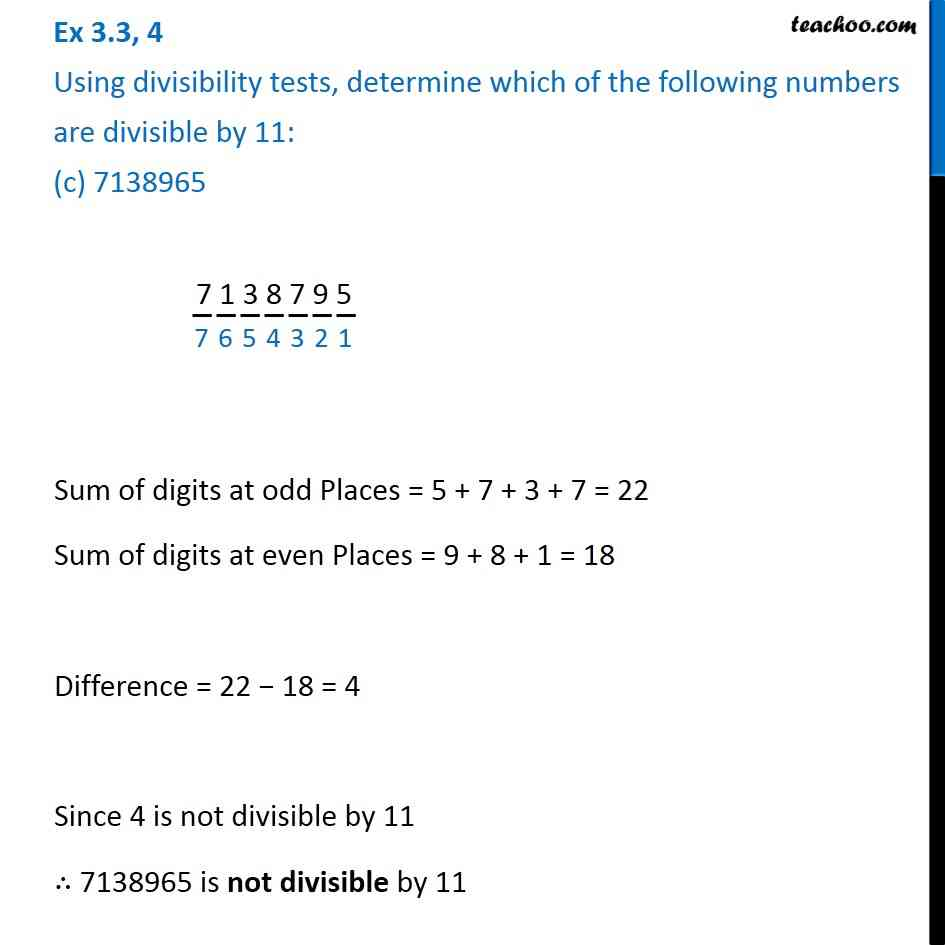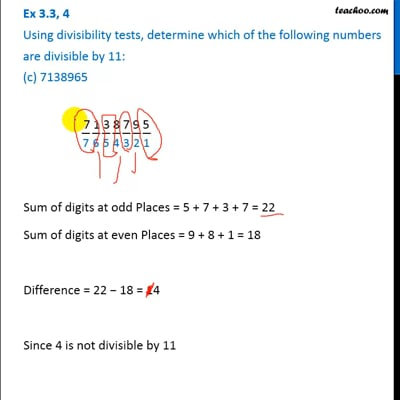Ex 3.3

Chapter 3 Class 6 Playing with Numbers
Serial order wiseThis video is only available for Teachoo black users

### Transcript

Ex 3.3, 4 Using divisibility tests, determine which of the following numbers are divisible by 11: (c) 7138965 7 1 3 8 7 9 5 Sum of digits at odd Places = 5 + 7 + 3 + 7 = 22 Sum of digits at even Places = 9 + 8 + 1 = 18 Difference = 22 − 18 = 4 Since 4 is not divisible by 11 ∴ 7138965 is not divisible by 11